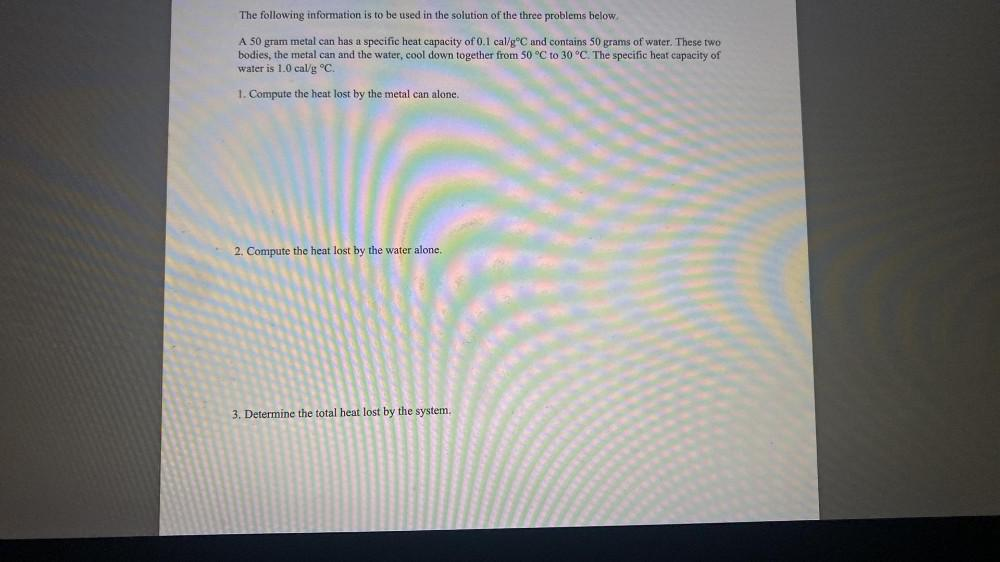Question:

# The following information is to be used in the solution of the three problems below. A 50 gram metal can has a specific heat capThe following information is to be used in the solution of the three problems below. A 50 gram metal can has a specific heat capacity of 0.1 cal/g °C and contains 50 grams of water. These two bodies, the metal can and the water, cool down together from 50 °C to 30 °C. The specific heat capacity of water is 1.0 cal/g °C. 1. Compute the heat lost by the metal can alone. 2. Compute the heat lost by the water alone. 3. Determine the total heat lost by the system.